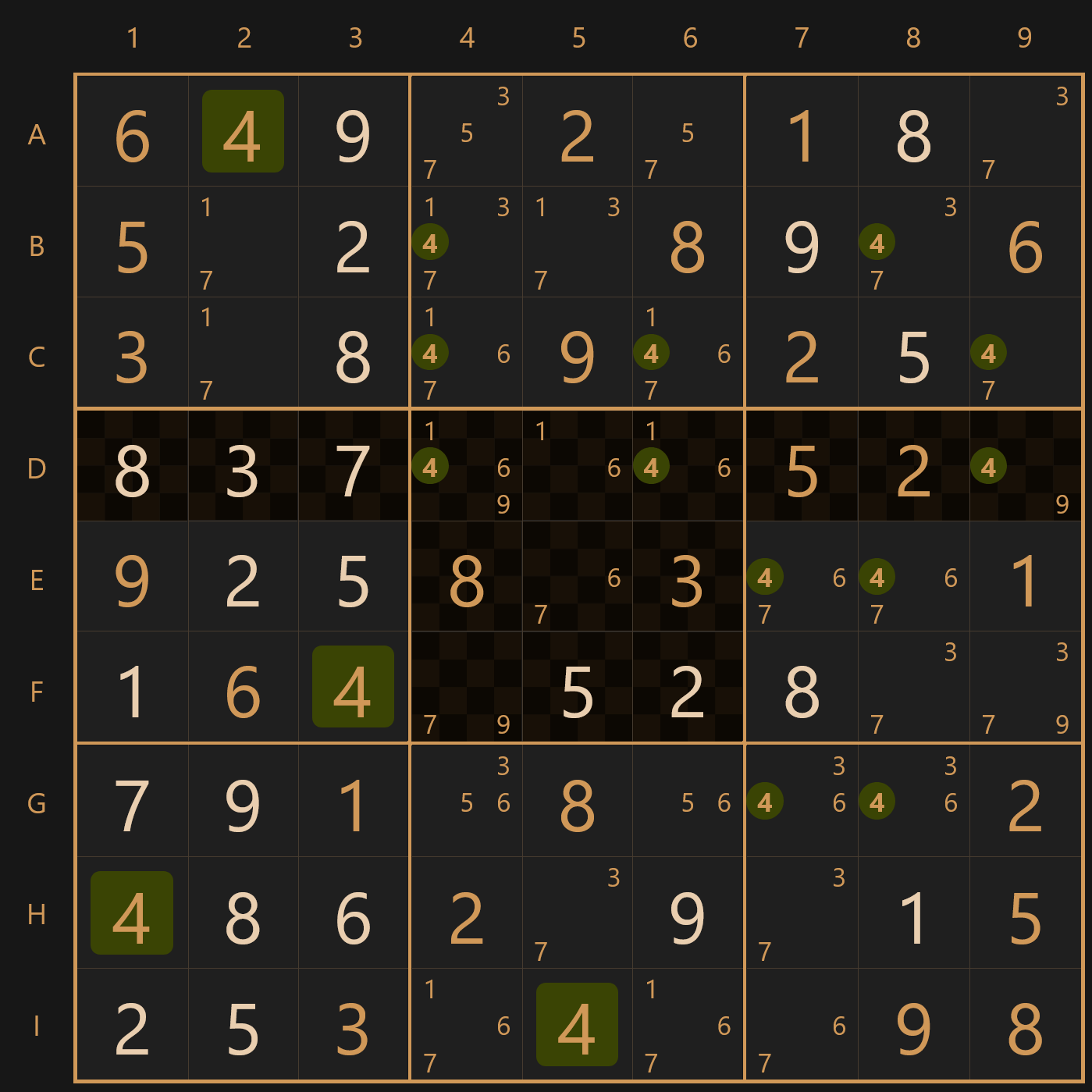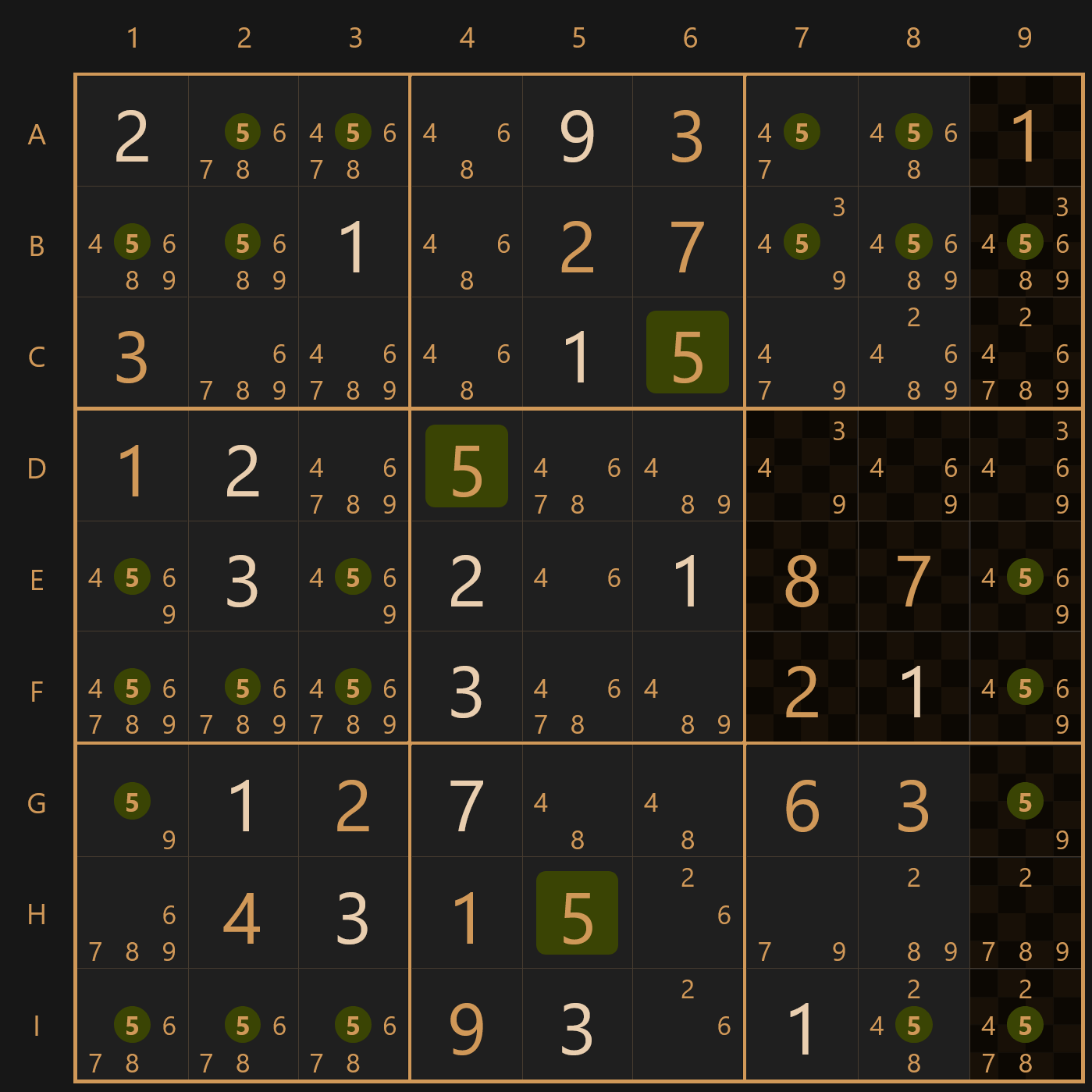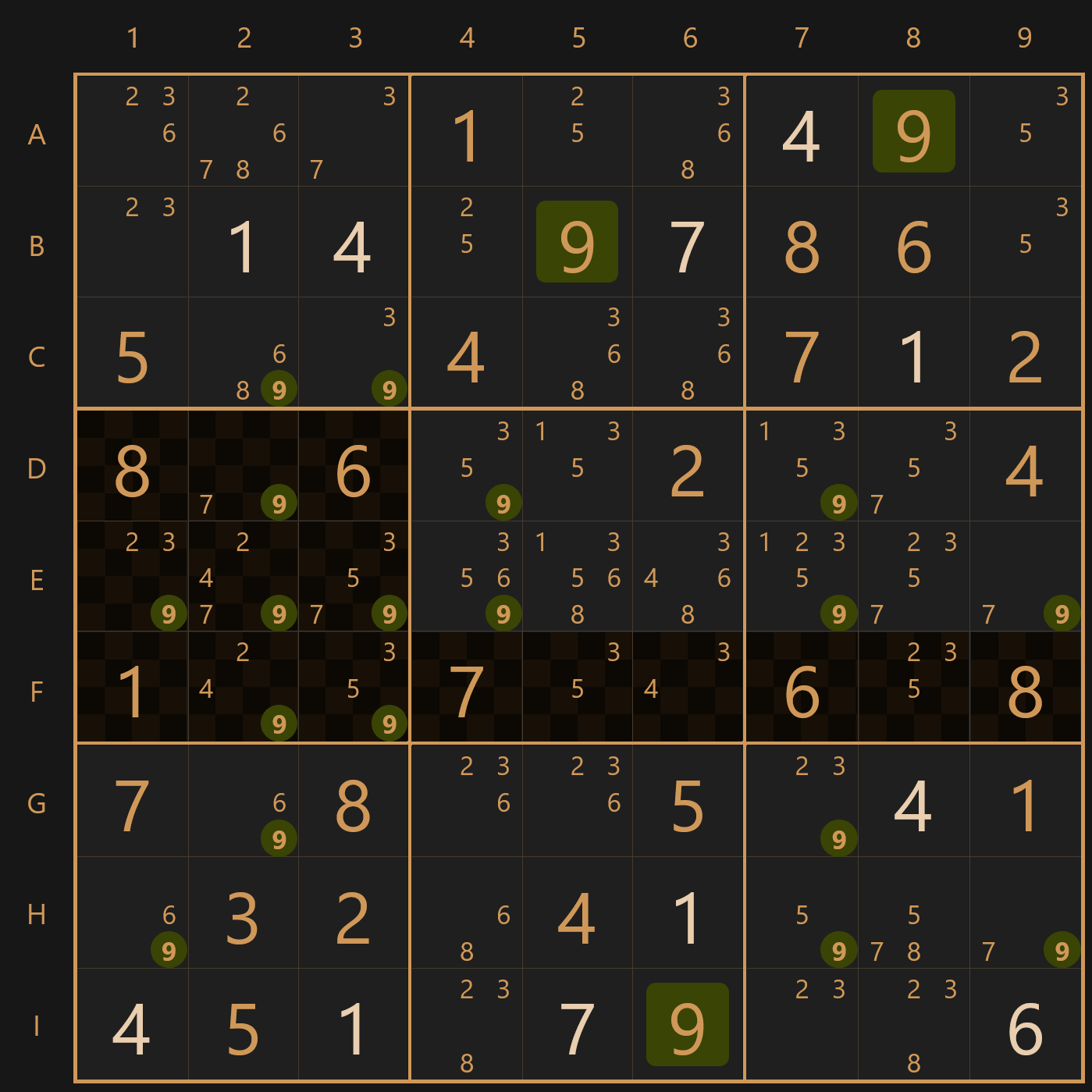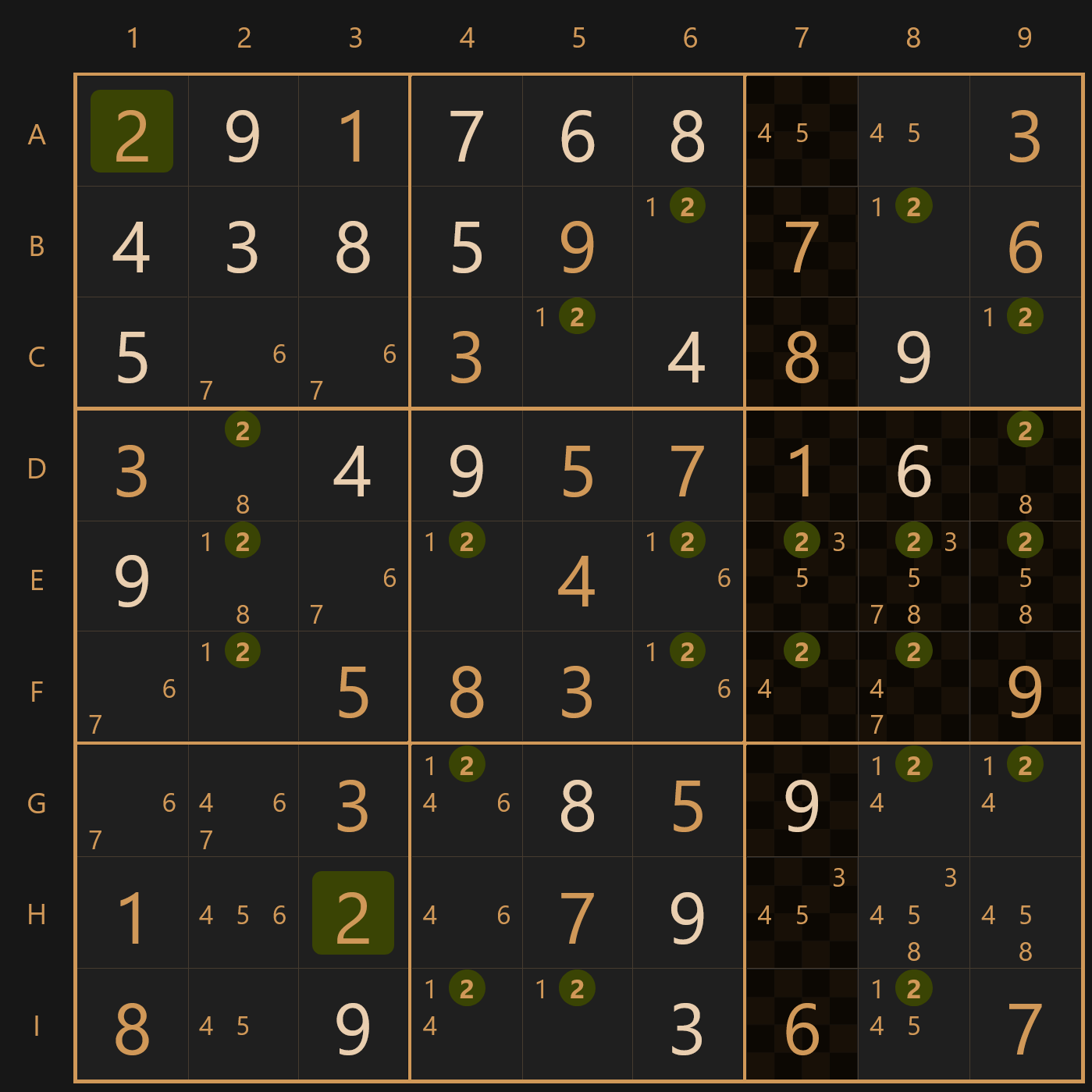# Hard sudoku puzzles @ thesudoku.app

In addition to the techniques described in easy and medium sudoku tutorials, to solve hard sudoku puzzles @ thesudoku.app, you will need to use additional solving techniques, that will help you reduce the number of pencil marks and solve puzzle step by step:

## Pointing pairs

If a candidate is present only in two or three cells of a 3x3 unit, and these cells belong to the same row or column, then this candidate cannot be a solution in other cells of this row or column respectively.

### Pointing pair in a rowFigure 1. Pointing pair in a row

### Pointing pair in a columnFigure 2. Pointing pair in a column

## Box/line reduction

This solving technique is very similar to the pointing pairs. If a candidate is present only in two or three cells of a row or column, and these cells belong to the same 3x3 unit, then this candidate cannot be a solution in other cells of this 3x3 unit.

### Box/line reduction in a rowFigure 3. Box/line reduction in a row

### Box/line reduction in a columnFigure 4. Box/line reduction in a column

This strategy is a logical extension of the nakes pairs and nakes triples solving techniques, although it is less common.

A «naked quad» is four cells from the same row, column or 3x3 unit, which have same four candidates left in different combinations. Since none of these candidates can be the solution in any other cell in this row, column or 3x3 unit respectively, you can remove pencil marks from the other cells.

## Hidden pairs

«Hidden pairs» solving technique is similar to naked pairs, except it's much harder to spot. If you feel stuck, you can use thesudoku.app smart hint feature.

If two candidates can be found only in two cells of the same row, column or 3x3 unit, then one of these candidates is the solution for one of these cells and the other candidate is the solution for the other cell. So you can eliminate the rest of the candidates from these cells